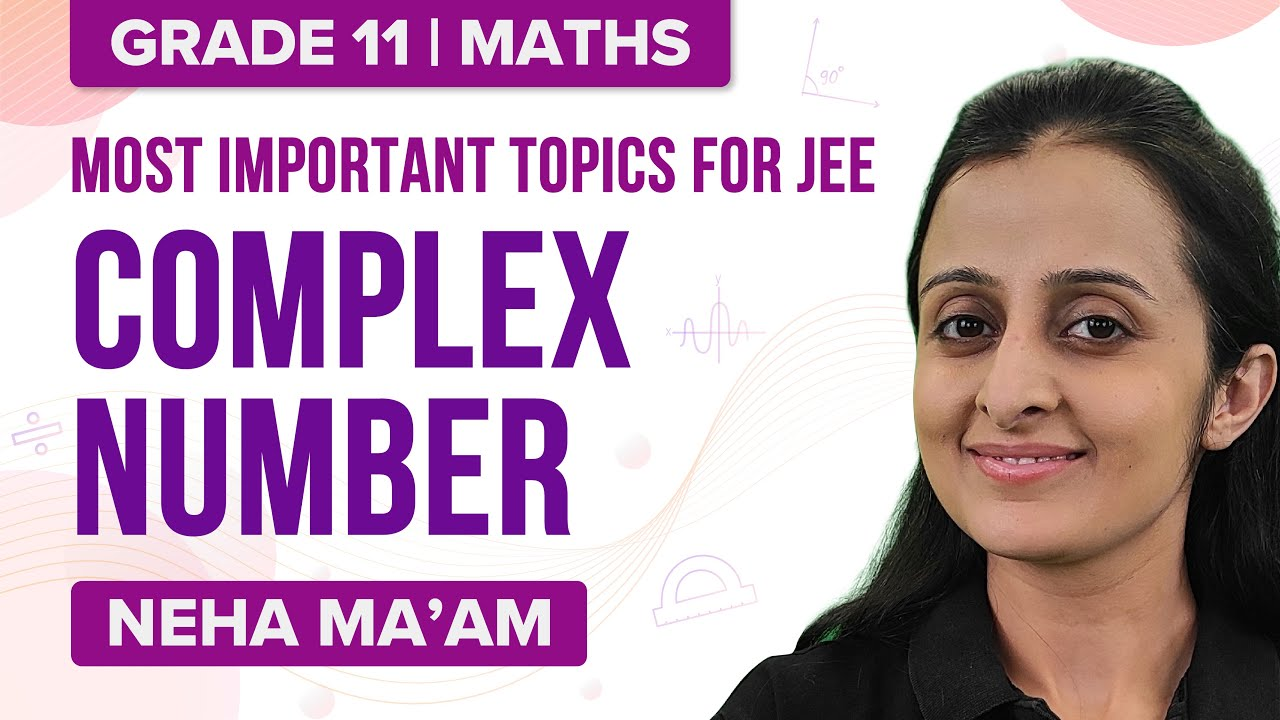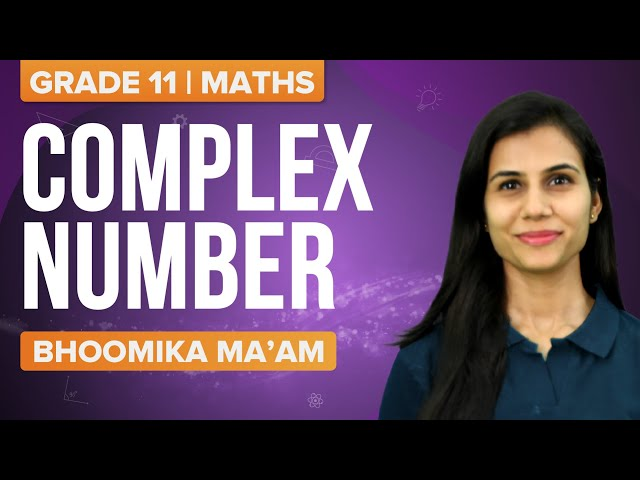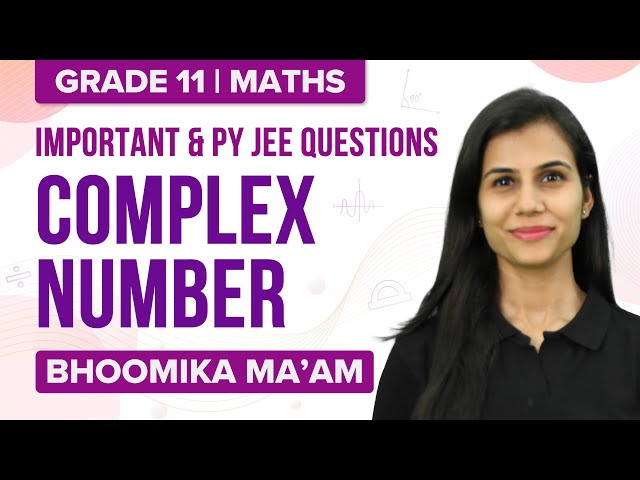Win up to 100% scholarship on Aakash BYJU'S JEE/NEET courses with ABNAT Win up to 100% scholarship on Aakash BYJU'S JEE/NEET courses with ABNAT

# JEE Advanced Maths Complex Numbers Previous Year Questions with Solutions

Students can find the JEE Advanced Maths complex numbers’ previous years’ questions and solutions on this page. These solutions are given in a step-by-step manner so that students can easily understand the problems. Practising previous years’ questions will help students to find out their weaker areas, and prepare for them better. Solutions are also available in PDF format for free. Students can easily download and revise the solutions offline.

Question 1: If 3/(2+ cos θ+i sin θ) = a+ib, then [(a-2)2+b2] is

(1) 0

(2) 1

(3) -1

(4) 2

Solution:

Given 3/(2+ cos θ+ i sin θ) = a+ib

3 ((2+ cos θ) – i sin θ)/(2+ cos θ + i sin θ)((2+cos θ)- i sin θ)

= ((6+ 3 cos θ) – i 3 sin θ)/((2+cos θ)2+ sin2 θ)

= ((6+ 3 cos θ) – i(3 sin θ))/(5+4 cos θ)

Comparing with a+ib, we get

a = (6+ 3 sin θ)/(5+ 4 cos θ)

b = -3 sin θ/(5+ 4 cos θ)

(a-2)2+b2 = [(6+ 3 sin θ)/(5+ 4 cos θ)]-2)2+(-3 sin θ/(5+ 4 cos θ))2

= (-4-5 cos θ)2+9 sin2θ)/(5+ 4 cos θ)2

= ((4+5 cos θ)2+9 sin2θ)/(5+ 4 cos θ)2

= (16 + 40 cos θ +25 cos2θ+ 9 sin2θ)/(5 +4 cos θ)2

= (16 + 40 cos θ+ 16 cos2θ+ 9 (sin2θ +cos2θ))/(5+ 4 cos θ)2

= (16 + 40 cos θ+ 16 cos2θ+ 9)/(5 +4 cos θ)2

= (16 cos2θ+ 40 cos θ+25)/(5+4 cos θ)2

= (4 cos θ+5)2/(5+4 cos θ)2

= 1

Hence option (2) is the answer.

Question 2: If z = x + iy is a complex number where x and y are integers. Then, the area of the rectangle whose vertices are the roots of the equation

$$\begin{array}{l}z\overline{z}^{3}+\overline{z}z^{3}=350\end{array}$$

(1) 48

(2) 32

(3) 40

(4) 80

Solution:

Given

$$\begin{array}{l}z\bar{z}^{3}+\bar{z}z^{3}=350\end{array}$$
$$\begin{array}{l}z\bar{z}(\bar{z}^{2}+z^{2})=350\end{array}$$

Take z = x+iy

(x+iy)(x-iy)[(x-iy)2+(x+iy)2] = 350

(x2+y2)(2x2-2y2) = 350

(x2+y2)(x2-y2) = 175 = (25)×7

x2+y2 = 25 (i)

x2-y2 = 7 ..(ii)

Adding (i) and (ii), we get

2x2 = 32

x = ±4

And y = ±3

Hence the vertices of the triangle are (4,3), (4, -3), (-4, -3) and (-4,3).

Area of rectangle = length × breadth

= 8×6

= 48

Hence option (1) is the answer.

Question 3: If 1, a1, a2,…an-1 are the nth roots of unity, then the value of (1-a1) (1 – a2)(1 – a3)…(1 – an-1) is

(1) √3

(2) 1/2

(3) n

(4) 0

Solution:

Given 1, a1, a2,…an-1 are the nth roots of unity.

So xn-1 = (x-1)(x-a1)…(x-an-1)

( xn-1) /(x-1) = (x-a1)(x-a2)…(x-an-1)

xn-1+xn-2+…x2+x+1 = (x-a1)(x-a2)…(x-an-1)

Substitute x = 1

(1-a1)(1-a2)…(1-an-1)

= 1+1+1+…n times

= n

Hence option (3) is the answer.

Question 4: If |(z+i)/(z-i)| = √3, then radius of the circle is

(1) 2/√21

(2) 1/√21

(3) √3

(4) √21

Solution:

Take z = x+iy

|z| = √(x2+y2)

Given |(z+i)/(z-i)| = √3

|(z+i) | = √3|(z-i)|

Put z = x+iy

|(x+(y+1)i) | = √3|(x+(y-1)i)|

Squaring we get

x2+ (y+1)2 = 3(x2+(y-1)2)

x2+ y2 +2y + 1 = 3x2+ 3y2– 6y +3

2x2+ 2y2– 8y + 2 = 0

x2+ y2– 4y + 1 = 0

Equation of circle is (x-h)2 + (y-k)2 = r2

Where (h, k) is the centre and radius is r.

(x-0)2 + (y-2)2 = √32

Hence option (3) is the answer.

Question 5: If α and β ∈ C are the distinct roots of the equation x2– x + 1 = 0, then α101+ β107 is equal to:

(1) 1

(2) 2

(3) -1

(4) 0

Solution:

x2– x +1 = 0

Solve by using quadratic formula, we get

x = (1±i√3)/2

= (1+i√3)/2 , (1-i√3)/2

= -(-1-i√3)/2, -(-1+i√3)/2

= -ω2, -ω

α= -ω2

β = -ω

α101+ β107 = (-ω2)101 + (-ω)107

= -(ω202+ ω107)

= -[(ω3)67 ω+ (ω3)35 ω2]

= -[ω +ω2]

= 1

Hence option (1) is the answer.

Question 6: The region represented by {z = x+iy ∈ C : z -Re(z) ≤1} is also given by the inequality: {z = x + iy ∈ C : z -Re(z) ≤ 1}

(1) y2 ≤ 2(x + 1/2)

(2) y2 ≤ x + (1/2)

(3) y2 ≥ 2(x + 1)

(4) y2 ≥ (x + 1)

Solution:

{z = x + iy ∈ C : z -Re(z) ≤ 1}

|z| = √(x2+y2)

Re(z) = x

z- Re(z) ≤1

=>√(x2+y2)-x≤ 1

=> √(x2+y2)≤ 1+x

=>(x2+y2)≤ (1+x2+2x)

=> y2 ≤ 2(x + 1/2)

Hence option (1) is the answer.

Question 7: If ((1+i)/(1-i))m/2 = ((1+i)/(i-1))n/3 = 1, (m, n∈N) then the greatest common divisor of the least values of m and n is :

Solution:

[(1+i)(1+i)/(1+i)(1-i)]m/2 = [(1+i)(-1-i)/(-1+i)(-1-i)]n/3 = 1 (i = √-1)

Solving LHS of above equation, we get

(2i/2)m/2 = 1

=> m = 8

Solving RHS,

[(-1-i-i+1)/(1+1)]n/3 = 1

(-2i/2)n/3 = 1

(-i)n/3 = 1

=> n = 12

Greatest common divisor of m and n is 4.

Question 8: If z is any complex number satisfying |z – 3 – 2i | ≤ 2, then the minimum value of |2z – 6 + 5i| is

(1) 2

(2) 1

(3) 3

(4) 5

Solution:

|z – 3 – 2i | ≤ 2

|2z – 6 + 5i| = 2|z – 3 + (5/2)i|

|z – 3 + (5/2)i|

=|z – 3 -2i+2i+(5/2)i| (using triangle inequality)

= |(z – 3 -2i)+(9/2)i|

≥ ||z – 3 -2i)|-(9/2)|

≥ |2-9/2|≥ 5/2

=> |z – 3 + (5/2)i|≥ 5/2

=> |2z – 6+ 5i|≥ 5

Hence option (4) is the answer.

Question 9: If z is a complex number such that the imaginary part of z is non-zero and a = z2 + z + 1 is real. Then, a cannot take the value

(1) -1

(2)1/3

(3) 1/2

(4) ¾

Solution:

Given a = z2+z+1

z2+z+1−a = 0

If solution is not real then b2-4ac<0.

1 – 4(1-a) <0

1 – 4 + 4a <0

-3 + 4a <0

4a <3

a< 3/4

Values of a less than 3/4 will give non real solutions.

Hence option (4) is the answer.

Question 10: Let a, b, x and y be real numbers such that a-b = 1 and y ≠ 0. If the complex number z = x + iy satisfies Im((az +b)/(z+1)) = y, then which of the following is(are) possible value(s) of x?

(1) -1 + √(1 – y2)

(2) -1 – √(1 – y2)

(3) 1 + √(1 + y2)

(4) 1 – √(1 + y2)

Solution:

a – b = 1

y ≠ 0

Im[(az +b)/(z+1)] = y

Let z = x+iy

=> Im [(a(x + iy) + b)/(x + iy + 1)][((x + 1) – iy)/((x + 1) – iy)] = y

=> [-(ax + b)y + ay(x+1)]/(x+1)2 + y2 = y

=> (-axy – by + axy + ay)/(x+1)2 + y2 = y

=> a – b = (x+1)2 + y2

=> 1 = (x+1)2 + y2

So x = -1 ±√(1-y2)

Hence option (1) and (2) are correct.

Complex Numbers and Quadratic Equations Previous Year Questions With Solution

## Complex Numbers Most Important Topics for JEE## Complex Numbers JEE Main Important Questions## Complex Numbers JEE Previous Year Questions